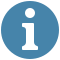## Number of islands visualization

Speed

Create islands#### Controls

Speed - increase or decrease the speed of the visualization

Islands- - create islands on the grid.
You can adjust the number of islands generated per click.
- You can also click on the grid cells to create an island.

Start - After adjusting the speed and creating islands, you can now start the visualization to see the workings of the algorithm.

Speed - If the grid becomes to cluttered you can reload it and repeat the above steps.

This is represents an empty area without any islands.

These are islands, which we are to count. The definition of an island is described in the algorithm section below.

This highlights how the algorithm traverses the grid looking fr islands, after the algorithm is complete, we have finished counting the number of islands.

### Number of islands

We are given a grid map of '1's and '0's(water). We are to count the number of islands on the grid. An island is surrounded by water and formed by connecting adjacent land horizontally or vertocally. In other words an island is a group of connected '1's. We assume all four edges of the whole grid are surrounded by water.

Algorithm

1. Mark the current node as visited(initially current node is the root node).

2. Check if current node is the goal, If so, then return it.

1. Iterate over children nodes of current node, and do the following:

2. Check if a child node is not visited.

1.If so, then, mark it as visited.

3. Go to it's sub tree recursively until you find the goal node(In other words, do the same steps here passing the child node as the current node in the next recursive call).

4. If the child node has the goal node in this sub tree, then, return it.

3. If goal node is not found, then goal node is not in the tree!

Computational complexity

This algorithm takes O(M + N) where M is the number of rows and E is the number of columns.

Applications

• topological sorting.
• bipartite graph testing.
• finding strongly connected components.
• solving puzzles that can only have one solution.
• detecting cycles in a graph.

References

number of islands